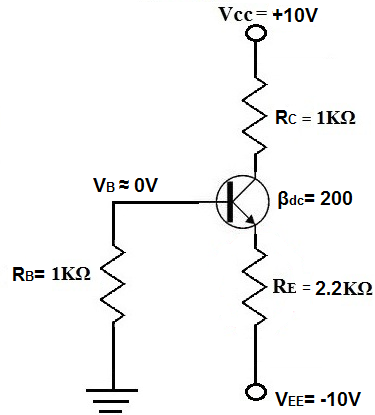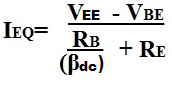﻿ Emitter Bias of a BJT Transistor# Emitter Bias of a BJT TransistorOne way to bias a BJT transistor is a method called emitter bias.

Emitter bias is a very good and stable way to bias transistors if both positive and negative power supplies are available. Emitter bias fluctuates very little with temperature variation and transistor replacement.

Below is a BJT transistor receiving emitter bias:You can see how that both positive and negative voltage supplies are necessary to bias a transistor in this way. Positive voltage is fed to the collector of the transistor and negative voltage is fed to the emitter.

### Calculations

Assuming this is a silicon transistor, the voltage drop across the base-emitter diode is equal to 0.7V.

To calculate the emitter current, IE, the formula is:So in the circuit above, the emitter current calculation is:

IE= (10V - 0.7V)/(2.2KΩ + (1KΩ/200))= 4.22mA

To calculate the collector voltage, VC, the formula is:

VC= VCC - ICRC = 10V - (4.23mA x 1KΩ) =5.77V

Again, emitter bias is an effective way to bias a BJT transistor. However, the voltage divider bias is still the most popular way to do so, while base bias is the least popular way because of the instability it provides if β changes.